Time
100

What do all of these shapes have in common?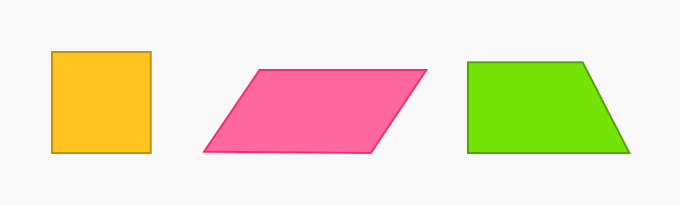a. quadrilaterals, 4 right angles, 2 pairs of parallel sides

b. quadrilaterals, 4 angles, 4 sides

b. quadrilaterals, 4 angles, 4 sides

100

What fraction is below?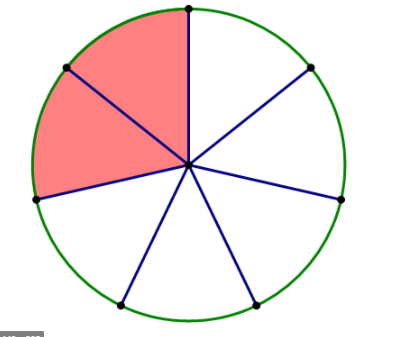2/7

100

Find the Perimeter.40 ft

100

What time is on the clock?10:00

200

Which shape has 4 right angles and 4 equal sides?

square

200

The circle is partitioned into ________.fourths

200

Find the area.60 cm2

200

What time does the clock show?5:45

300

a. trapezoid

b. rhombus

c. triangle

d. rectangle

c. triangle

300

What fraction of the circle is shaded?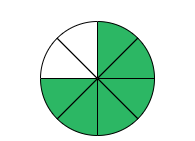6/8

300

What is the perimeter of this rectangle?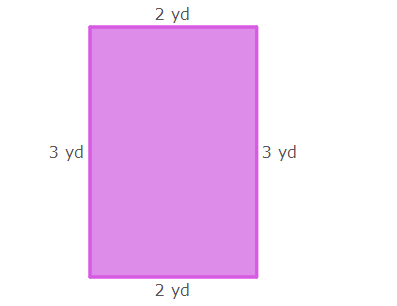10 yards (2+3+2+3)

300

What time is shown on the clock?11:19

400

What quadrilaterals have 4 right angles?

a. square and rhombus

b. square and rectangle

c. square and trapezoid

b. square and rectangle

400

What fraction is represented on this number line?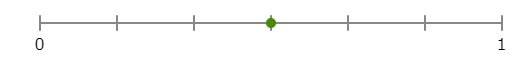3/6

400

What is the area of this rectangle?6 square yards (2x3)

4001:15

500

What is a polygon with 4 sides?

a. triangle

b. pentagon

500

Which number below will make the inequality true?      ?/5 < 4/5

a. 5

b. 2

c. 4

b. 2

500

Find the Perimeter.22 cm

500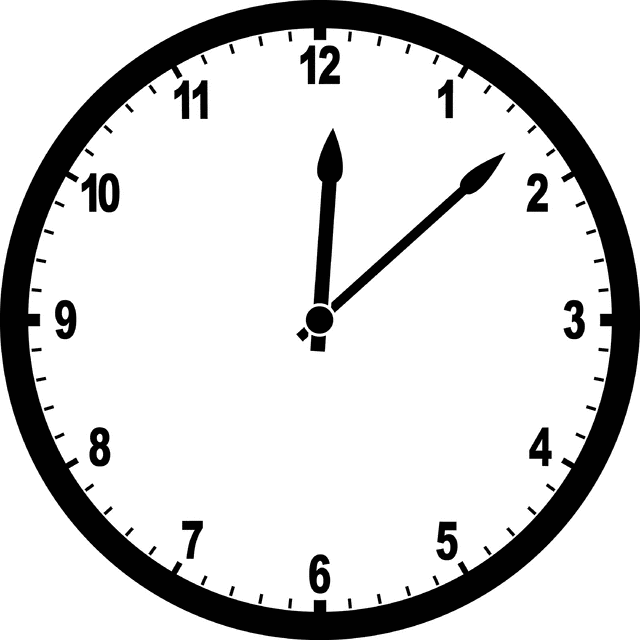12:08

Click to zoom